## Smith Normal Form

On the first day of class we learned about Smith normal forms of matrices.
> A := Matrix(2,2,[1,2,3,4]);
> A;
[1 2]
[3 4]
> SmithForm(A);
[1 0]
[0 2]

[ 1  0]
[-1  1]

[-1  2]
[ 1 -1]

As you can see, computed the Smith form, which is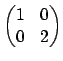. What are the other two matrices it output? To see what any command does, type the command by itself with no arguments followed by a semicolon.
> SmithForm;
Intrinsic 'SmithForm'

Signatures:

(<Mtrx> X) -> Mtrx, AlgMatElt, AlgMatElt
[
k: RngIntElt,
NormType: MonStgElt,
Partial: BoolElt,
RightInverse: BoolElt
]

The smith form S of X, together with unimodular matrices
P and Q such that P * X * Q = S.

As you can see, SmithForm returns three arguments, a matrix and matrices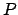and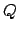that transform the input matrix to Smith normal form. The syntax to receive'' three return arguments is natural, but uncommon in other programming languages:
> S, P, Q := SmithForm(A);
> S;
[1 0]
[0 2]
> P;
[ 1  0]
[-1  1]
> Q;
[-1  2]
[ 1 -1]
> P*A*Q;
[1 0]
[0 2]

Next, let's test the limits. We make a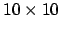integer matrix with entries between 0 and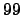, and compute its Smith normal form.
> A := Matrix(10,10,[Random(100) : i in [1..100]]);
> time B := SmithForm(A);
Time: 0.000

Let's print the first row of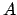, the first and last row of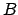, and the diagonal of:
> A;
( 4 48 84  3 58 61 53 26  9  5)
> B;
(1 0 0 0 0 0 0 0 0 0)
> B;
(0 0 0 0 0 0 0 0 0 51805501538039733)
> [B[i,i] : i in [1..10]];
[ 1, 1, 1, 1, 1, 1, 1, 1, 1, 51805501538039733 ]

Let's see how big we have to makein order to slow down . These timings below are on a 1.6Ghz Pentium 4-M laptop running Magma V2.11 under VMware Linux. I tried exactly the same computation running Magma V2.17 natively under Windows XP on the same machine, and it takes twice as long to do each computation, which is strange.
> n := 50; A := Matrix(n,n,[Random(100) : i in [1..n^2]]);
> time B := SmithForm(A);
Time: 0.050
> n := 100; A := Matrix(n,n,[Random(100) : i in [1..n^2]]);
> time B := SmithForm(A);
Time: 0.800
> n := 150; A := Matrix(n,n,[Random(100) : i in [1..n^2]]);
> time B := SmithForm(A);
Time: 4.900
> n := 200; A := Matrix(n,n,[Random(100) : i in [1..n^2]]);
> time B := SmithForm(A);
Time: 19.160


can also work with finitely generated abelian groups.

> G := AbelianGroup([3,5,18]);
> G;
Abelian Group isomorphic to Z/3 + Z/90
Defined on 3 generators
Relations:
3*G.1 = 0
5*G.2 = 0
18*G.3 = 0
> #G;
270
> H := sub<G | [G.1+G.2]>;
> #H;
15
> G/H;
Abelian Group isomorphic to Z/18


William Stein 2004-05-06